## PARALLEL DIRECT METHOD OF LES FOR TURBULENT WIND FIELD WITH HIGH REYNOLDS NUMBER 1)

School of Aeronautics and Astronautics, Sun Yat-Sen University, Guangzhou 510275,China

 基金资助: 1)国家自然科学基金.  11772362

Received: 2020-02-21   Accepted: 2020-04-13   Online: 2020-05-18Abstract

In the environmental fluid mechanics, the turbulent wind field in the atmospheric layer is the driving force and the foundation for the study of the natural environment characteristics such as the wind and sand flow and the wind and snow flow. The calculation simulation of the turbulent wind field is usually used the turbulent boundary layer model, where the large eddy simulation (LES) is an effective computational tool. The parallel computing technology is one of the key technologies for the LES simulation to solve the large-scale turbulent wind field with the high Reynolds number. In the LES simulation of the turbulent wind field, the parallel computing technique of the pressure Poisson equation is the difficult point for the scale parallel calculation. Based on the characteristics of the turbulent wind field flow simulation, the horizontal grids are equidistant spacing and the grids perpendicular to the ground are non-equidistant. Using the FFT decoupling three-dimensional Poisson equation to make it a one-dimensional three-dimensional diagonal equation, and the parallel-able PDD technology is used to solve the three-diagonal equation for solving the difficult problem of the pressure Poisson equation in the scale parallel calculation, and the three-dimensional pressure Poisson equation can be solved directly in parallel. Combined with the other parallel momentum equation calculations, the parallel direct method of LES (PDM-LES) for the turbulent wind field simulation is established. The new method is tested in parallel on the supercomputer, and the parallel computing efficiency is 90${\%}$. The new method can be used for the large-scale parallel calculation for LES simulation of the turbulent wind field. The results show that there is a striped pre-order structure near the wall in the transient velocity distribution. The velocity distribution of the average field conforms to the logarithmic law, and the turbulence characteristic of the wind field is basically reasonable.

Keywords： wind field ; wall-bounded model ; high Reynolds number ; parallel computations

Bao Yun, Xi Lingchu. PARALLEL DIRECT METHOD OF LES FOR TURBULENT WIND FIELD WITH HIGH REYNOLDS NUMBER 1). Chinese Journal of Theoretical and Applied Mechanics[J], 2020, 52(3): 656-662 DOI:10.6052/0459-1879-20-052

## 1 大涡模拟控制方程和亚网格模式

$\dfrac{\partial u_i}{\partial x_i}=0 \\ \dfrac{\partial u_i}{\partial t}+u_j \dfrac{\partial u_i}{\partial x_j} =-\dfrac{\partial P}{\partial x_i}+ \dfrac 1{Re} \dfrac{\partial^2 u_i}{\partial x_i\partial x_j} +\dfrac{\partial \tau_{ij}}{\partial x_j}$

LES方程和NS方程的不同之处是LES方程包含了亚网格通量. 这些亚网格通量需要建立封闭模式,是大涡模拟的关键问题之一. 关于复杂湍流中的亚网格通量模式仍在继续研究和发展中,目前最常用的模式是涡黏和 涡扩散模型. Smagorinsky涡黏模式是最早提出的亚网格模式,并被工业界广泛应用. 涡黏模式的公式为

$\tau_{ij}=2 v_t \bar S_{ij} +\dfrac 13 \tau_{kk} \delta_{ij}$

$v_t=C^2_s \varDelta^2 | \bar S |$

$C_{s}$是模式系数, $\varDelta$是网格尺度,$| \bar S | =(2 \bar S_{ij} \bar S_{ij})^{1/2}$, 而 $\bar S_{ij}=(\partial \bar u_i/\partial x_j+ \partial \bar u_j / \partial x_i)/2$是可解尺度速度变形率张量. 各项同性湍流理论可确定模式系数$C_{s} =0.10$. 但在实际应用中发现,Smagorinsky涡黏模式在底边界壁面附近耗散过大,需要调整模式系数. 本文将采用Smagorinsky涡黏模式作为亚网格模式进行大涡模拟计算.

## 2 不可压流动LES模拟的并行直接求解方法

### 图1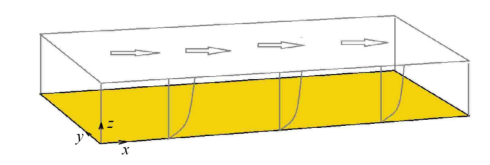Fig.1   The wind field calculation domain schematic

$\dfrac{\partial^2 P}{\partial x^2}+\dfrac{\partial^2 P}{\partial y^2}+\dfrac{\partial^2P}{\partial z^2}=d$

$\dfrac{\partial P}{\partial n} \Big |_{\hbox{边界}}=0$

$\dfrac{P_{i-1,j,k}-2P_{i,j,k}+P_{i+1,j,k}}{\Delta x^2}+ \dfrac{P_{i,j-1,k}-2P_{i,j,k}+P_{i,j+1,k}}{\Delta y^2}+\\ \qquad \dfrac{2P_{i,j,k+1}}{(\Delta z_{k+1}+\Delta z_k)\Delta z_k}+ \dfrac{2P_{i,j,k-1}}{(\Delta z_{k-1}+\Delta z_k)\Delta z_k}-\\ \qquad \dfrac{2P_{i,j,k}}{\Delta z_k} \Big ( \dfrac 1{\Delta z_{k+1}+\Delta z_k}+\dfrac 1 {\Delta z_{k-1}+\Delta z_k } \Big ) =d_{i,j,k}$

$\hat P_{p,q,k}=\dfrac 1{\sqrt{4MN}} \sum\limits^{M-1}_{i=0} \sum\limits^{N-1}_{j=0} 4P_{i,j,k}\cos \dfrac{\pi (i+1/2)p}M \cdot \\ \qquad \cos \dfrac{\pi(j+1/2)q}N$

$M$对应$x$方向的网格数$N_x$,$N$对应$y$方向的网格数$N_y$. 以上变换可以通过使用FFTW 软件包实现. 将上式变换应用到压力泊松方程中,可得到沿$z$方向联立的三对角方程

$a_{p,q,k}\cdot \hat P_{p,q,k-1}+b_{p,q,k}\cdot \hat P_{p,q,k}+c_{p,q,k}\cdot \hat p_{p,q,k+1}=\hat d_{p,q,k}$

### 2.2 并行计算规模和计算效率

Table 1  The computational efficiency of different compute nodes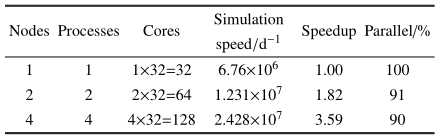## 3 三维风场的规模并行计算

### 图2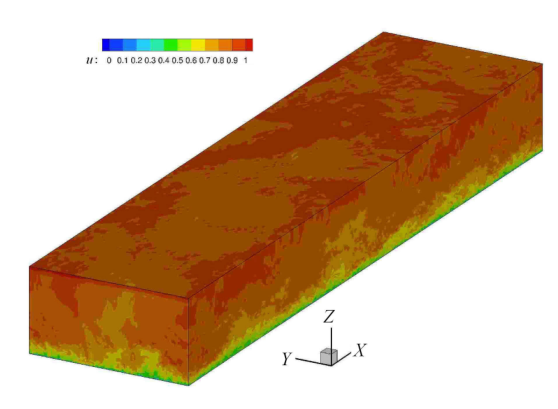Fig.2   3D instantaneous speed cloud map

### 图3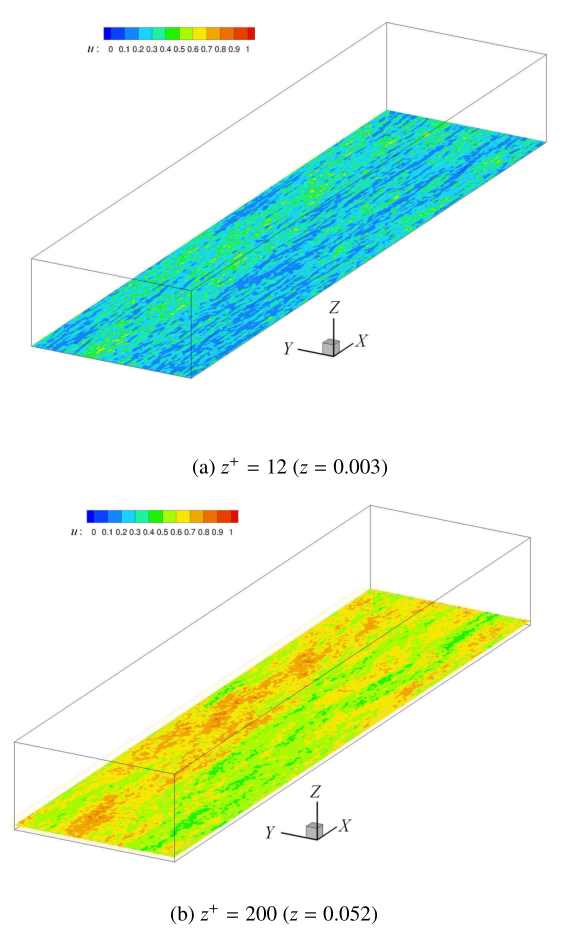Fig.3   Instant velocity distribution of ${xy}$ planes at different altitudes

### 图4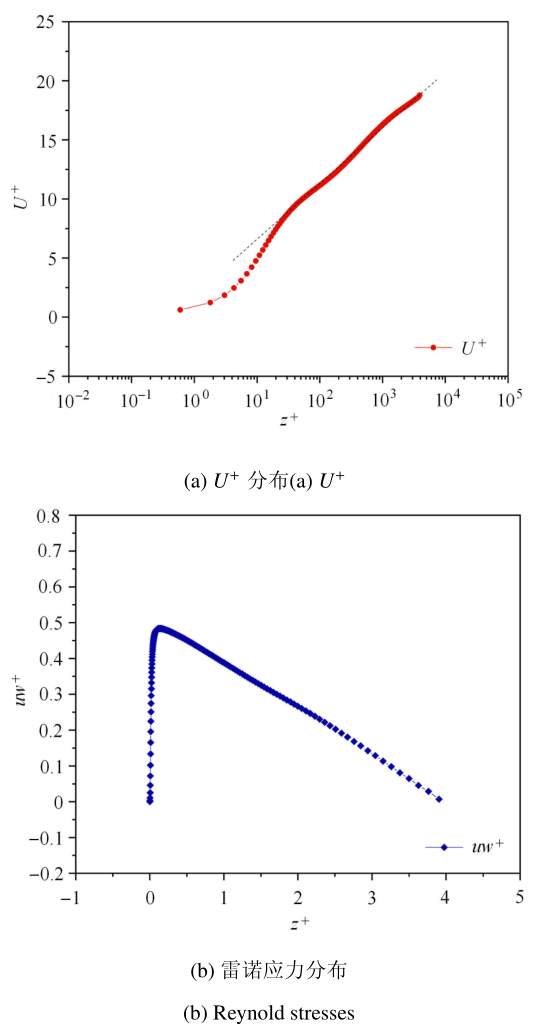Fig.4   Average velocity field characteristics of wall turbulence

## 4 结论

(1) 本文将高效的并行直接求解方法扩展到三维壁湍流风场的大涡模拟计算中,建立了三维壁湍流风场大涡模拟的高效并行直接求解方法PDM-LES.

(2) 对并行直接求解方法的并行计算效率进行的验证计算结果表明,在"天河二号"超级计算机上进行的1至4个节点,最大用到128核的并行计算效率达到90${\%}$.

(3) 计算${Re}=10 000$的结果显示,湍流风场瞬时速度分布在近壁区存在条带状的拟序结构,并远离壁面条带结构尺度增加. 平均场的速度分布符合湍流速度分布特性,即近壁面在$z^{ + } \approx 20$之内$U^{ + }$为线性分布,在外区$U^{ +}$满足对数律分布. 计算得到的风场湍流特性基本合理.

/

 〈〉